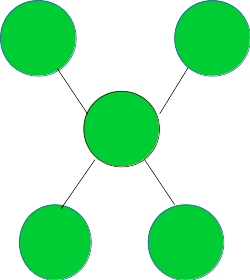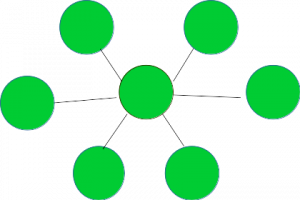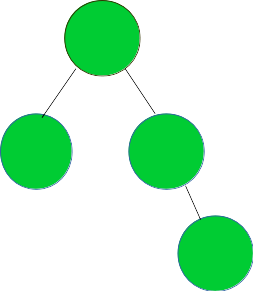# Check if the given graph represents a Star Topology

Given a graph G, the task is to check if it represents a Star Topology.

A Star Topology is the one shown in the image below:Examples:

```Input : Graph =Output : YES

Input : Graph =Output : NO
```

## Recommended: Please try your approach on {IDE} first, before moving on to the solution.

A graph of V vertices represents a star topology if it satisfies the following three conditions:

1. One node (also called the central node) has degree V – 1.
2. All nodes except the central node have degree 1.
3. No of edges = No of Vertices – 1.

The idea is to traverse the graph and check if it satisfies the above three conditions. If yes, then it represents a Star Topology.

Below is the implementation of the above approach:

 `// CPP program to check if the given graph ` `// represents a Star Topology ` `#include ` ` `  `using` `namespace` `std; ` ` `  `// A utility function to add an edge in an ` `// undirected graph. ` `void` `addEdge(vector<``int``> adj[], ``int` `u, ``int` `v) ` `{ ` `    ``adj[u].push_back(v); ` `    ``adj[v].push_back(u); ` `} ` ` `  `// A utility function to print the adjacency list ` `// representation of graph ` `void` `printGraph(vector<``int``> adj[], ``int` `V) ` `{ ` `    ``for` `(``int` `v = 0; v < V; ++v) { ` `        ``cout << ``"\n Adjacency list of vertex "` `             ``<< v << ``"\n head "``; ` `        ``for` `(``auto` `x : adj[v]) ` `            ``cout << ``"-> "` `<< x; ` `        ``printf``(``"\n"``); ` `    ``} ` `} ` ` `  `/* Function to return true if the graph represented  ` `   ``by the adjacency list represents a Star topology  ` `   ``else return false */` `bool` `checkStarTopologyUtil(vector<``int``> adj[], ``int` `V, ``int` `E) ` `{ ` ` `  `    ``// Number of edges should be equal ` `    ``// to (Number of vertices - 1) ` `    ``if` `(E != (V - 1)) ` `        ``return` `false``; ` ` `  `    ``// a single node is termed as a star topology ` `    ``// having only a central node ` `    ``if` `(V == 1) ` `        ``return` `true``; ` ` `  `    ``int``* vertexDegree = ``new` `int``[V + 1]; ` `    ``memset``(vertexDegree, 0, ``sizeof` `vertexDegree); ` ` `  `    ``// calculate the degree of each vertex ` `    ``for` `(``int` `i = 1; i <= V; i++) { ` `        ``for` `(``auto` `v : adj[i]) { ` `            ``vertexDegree[v]++; ` `        ``} ` `    ``} ` ` `  `    ``// countCentralNodes strores the count of nodes  ` `    ``// with degree V - 1, which should be equal to 1 ` `    ``// in case of star topology ` `    ``int` `countCentralNodes = 0, centralNode = 0; ` ` `  `    ``for` `(``int` `i = 1; i <= V; i++) { ` `        ``if` `(vertexDegree[i] == (V - 1)) { ` `            ``countCentralNodes++; ` `            ``// Store the index of the central node ` `            ``centralNode = i; ` `        ``} ` `    ``} ` ` `  `    ``// there should be only one central node ` `    ``// in the star topology ` `    ``if` `(countCentralNodes != 1) ` `        ``return` `false``; ` ` `  `    ``for` `(``int` `i = 1; i <= V; i++) { ` `        ``// except for the central node  ` `        ``// check if all other nodes have ` `        ``// degree 1, if not return false ` `        ``if` `(i == centralNode) ` `            ``continue``; ` `        ``if` `(vertexDegree[i] != 1) { ` `            ``return` `false``; ` `        ``} ` `    ``} ` ` `  `    ``// if all three necessary ` `    ``// conditions as discussed, ` `    ``// satisfy return true ` `    ``return` `true``; ` `} ` ` `  `// Function to check if the graph  ` `// represents a Star topology ` `void` `checkStarTopology(vector<``int``> adj[], ``int` `V, ``int` `E) ` `{ ` `    ``bool` `isStar = checkStarTopologyUtil(adj, V, E); ` `    ``if` `(isStar) { ` `        ``cout << ``"YES"` `<< endl; ` `    ``} ` `    ``else` `{ ` `        ``cout << ``"NO"` `<< endl; ` `    ``} ` `} ` ` `  `// Driver code ` `int` `main() ` `{ ` `    ``// Graph 1 ` `    ``int` `V = 5, E = 4; ` `    ``vector<``int``> adj1[V + 1]; ` `    ``addEdge(adj1, 1, 2); ` `    ``addEdge(adj1, 1, 3); ` `    ``addEdge(adj1, 1, 4); ` `    ``addEdge(adj1, 1, 5); ` `    ``checkStarTopology(adj1, V, E); ` ` `  `    ``// Graph 2 ` `    ``V = 5, E = 4; ` `    ``vector<``int``> adj2[V + 1]; ` `    ``addEdge(adj2, 1, 2); ` `    ``addEdge(adj2, 1, 3); ` `    ``addEdge(adj2, 3, 4); ` `    ``addEdge(adj2, 4, 5); ` `    ``checkStarTopology(adj2, V, E); ` ` `  `    ``return` `0; ` `} `

Output:

```YES
NO
```

Time Complexity: O(V + E) where V and E are the numbers of vertices and edges in the graph respectively.

My Personal Notes arrow_drop_upCheck out this Author's contributed articles.

If you like GeeksforGeeks and would like to contribute, you can also write an article using contribute.geeksforgeeks.org or mail your article to contribute@geeksforgeeks.org. See your article appearing on the GeeksforGeeks main page and help other Geeks.

Please Improve this article if you find anything incorrect by clicking on the "Improve Article" button below.